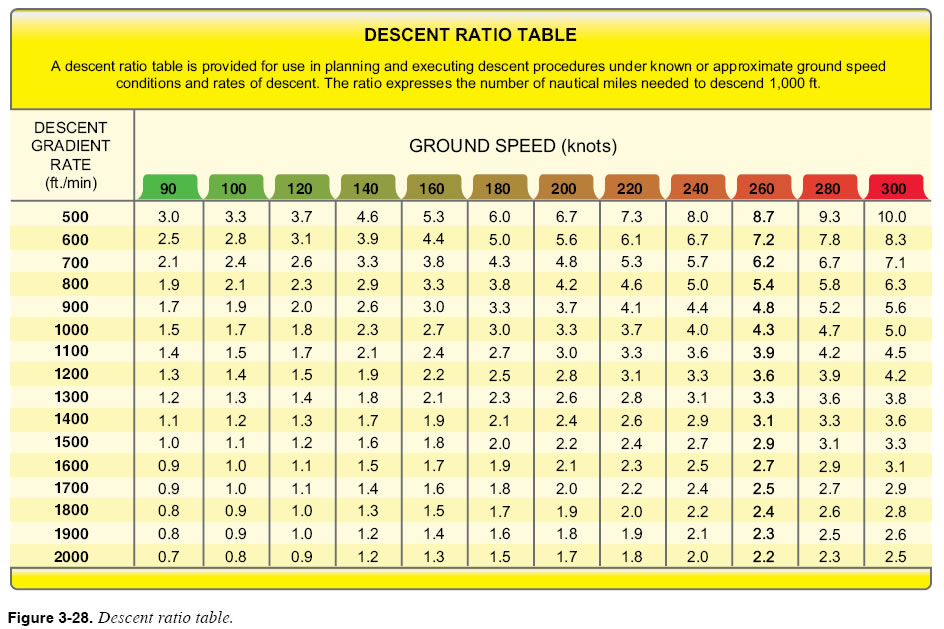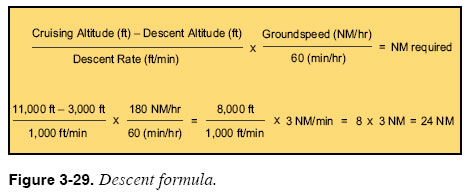CHAPTER 3. Navigation Manual Descent Calculations The simplest technique for calculating the distance required to descend uses a descent ratio. The table in Figure 3-28 lists a descent ratio for many combinations of planned descent speeds and descent rates. Calculating a descent is a simple matter of looking up the descent ratio for your target descent rate and groundspeed, and multiplying the descent ratio by the number of thousands of feet in altitude that you must descend. For example, suppose you are asked to descend from 11,000 feet to meet a crossing restriction at 3,000 feet. Since there is a 200-knot speed restriction while approaching the destination airport, you choose a descent speed of 190 knots and a descent rate of 1,000 feet per minute (fpm). Assuming a 10-knot headwind component, groundspeed in the descent is 180 knots. Referring to the table in Figure 3-28, the planned descent speed and rate indicate a ratio of 3.0. This means that you will need 3 NM for every 1,000 feet of descent. You must descend a total of 8,000 feet (11,000 feet – 3,000 feet). A total of 24 NM is needed to descend 8,000 feet (3 NM × 8 = 24 NM), and must, therefore, begin the descent 24 NM away from the end-of-descent point.Another technique for calculating descents is to use the formula shown in Figure 3-29. A descent table can be found in the front of each set of U.S. Terminal Procedures on page D-1. Working through the formula for the ECA VOR crossing restriction example, 8 minutes is needed to descend 8,000 feet at the planned descent rate of 1,000 fpm. At your planned descent speed of 180 knots, you will cover 3 NM per minute. Thus, in 8 minutes, you will cover 24 NM. Once again, you must start the descent 24 NM prior to ECA to meet the crossing restriction.